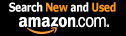CTK Exchange Front Page Movie shortcuts Personal info Awards Reciprocal links Terms of use Privacy Policy Cut The Knot! MSET99 Talk Games & Puzzles Arithmetic/Algebra Geometry Probability Eye Opener Analog Gadgets Inventor's Paradox Did you know?... Proofs Math as Language Things Impossible My Logo Math Poll Other Math sit's Guest book News sit's Recommend this site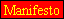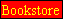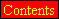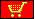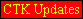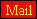CTK Exchange

 Subject: "Wythoff Array, Pythagorean Triplets, Primes" Previous Topic | Next Topic
 ConferencesThe CTK ExchangeThis and thatTopic #745Printer-friendly copyEmail this topic to a friend Reading Topic #745
Kenneth Ramseyguest
Jan-06-07, 06:50 PM (EST)

"Wythoff Array, Pythagorean Triplets, Primes"

 The Wythoff Array (https://www.research.att.com/~njas/sequences/A035513) is a square array of Fibonacci like sequences T(i,j) = T(i,j-1) + T(i,j-2) where T(i,1) = i and T(i,2) = Floor(i*(sqrt(5)+1)/2). Each row of the Wythoff Array has a characteristic number A(i) which is a constant that is equal to value of T(i,2n)^2 - T(i,2n-1)*T(i,2n+1). If the index n is off by one, i.e. even-odd-even instead of odd-even-odd we get A(i) = T(i,2n)*T(i,2n+2) - T(i,2n+1)^2 The sequence A(i) appears to be identical to that at https://www.research.att.com/~njas/sequences/A022344 althoughI dont have a proof. No more than two rows share the same characteristic and the second row is just the extension of the sequence in the first row T(i,j) beginning with the pair which is extended until the two terms are reached.Now Horadam has previously published a result concerning Fibonnacci series and Pythagorean triples "Fibonacci Number Triples" Amer. Math. Monthly 68(1961) 751-753. That paper shows that if F(0), F(1), F(2) and F(3) are 4 sequential numbers of a Fibonacci type sequence then P = (2F(1)*F(2),F(0)*F(3),2F(1)F(2)+F(0)^2) is a Pythagorean triplet.That is (2F(1)*F(2))^2 + (F(0)*F(3))^2 = (2F(1)*F(2)+F(0)^2)^2.I checked as to what sequence I would get if H(i) = T(i,j)^2 + 2(T(i,j+1)*T(i,j+2). In each case the sequence is a bijection of a primitive Fibonnacci sequence F(i) multiplied by G where G is the greatest common factor of any two adjacent terms, that is H(i) =G*F(2i-1). By filling in the intermediate values F(2i) = (H(i+1)-H(i))/G it is possible to compute the characteristic values C(i) of these new Fibonacci sequence. Surprisingly, I got the following sequence:1,1,1,1,1,121,361,1,961,361,961,1,841,121,1,... These terms are all 1 or the square of a prime ending in 1 or 9. After only 54 terms the squares of all such primes from 11 to 149 have been included and no composite term included except the square of such a prime. Do you find this to be a coincidence or a remarkable discovery?

Alert | IP Printer-friendly page | Reply | Reply With Quote | Top
Pierre Charland
Member since Dec-22-05
Jan-09-07, 10:40 PM (EST)1. "RE: Wythoff Array, Pythagorean Triplets, Primes"
In response to message #0

 Looking in my notes for Wythoff array, I found Stolarsky array.I don't konw the relation between those sequences,which are almost identical.A035513,Wythoff array read by antidiagonals,1, 2, 4, 3, 7, 6, 5, 11, 10, 9, 8, 18, 16, 15, 12, 13, 29, 26, 24,..as a square array:1 2 3 5 8 13 21 34 55 89 144 4 7 11 18 29 47 76 123 199 322 6 10 16 26 42 68 110 178 288 9 15 24 39 63 102 165 267 12 20 32 52 84 136 220 14 23 37 60 97 157 17 28 45 73 118 19 31 50 81 22 36 58 25 41 27 A035506, Stolarsky array read by antidiagonals,1, 2, 4, 3, 6, 7, 5, 10, 11, 9, 8, 16, 18, 15, 12, 13, 26, 29, 24,..as a square array:1 2 3 5 8 13 21 34 55 89 4 6 10 16 26 42 68 110 178 7 11 18 29 47 76 123 199 9 15 24 39 63 102 165 12 19 31 50 81 131 14 23 37 60 97 17 28 45 73 20 32 52 22 36 25 Ref:"A New Numerical Triangle Showing Links With Fibonacci Numbers",Fibonacci Quartely, nov 1991, p.316-321.AlphaChapMtl

Alert | IP Printer-friendly page | Reply | Reply With Quote | TopKenneth Ramseyguest
Jan-10-07, 11:29 PM (EST)

2. "RE: Wythoff Array, Pythagorean Triplets, Primes"
In response to message #1

 Yes. The arrays are very similar and my observation holds also for the Stolarsky array only the sequence order is different.In each case the Pythagorean Hypotenus Fibonacci sequence is defined by c = P(2i+1) = (2F(i,j+1)*F(i,j+2)/G + F(i,j)^2/G where G is the greatest common divisor. To get the even terms of the sequence simply take the difference of the adjacent odd terms. For each Fibonacci sequence the characteristic value is defined by |F(i-1)*F(i+1)-F^i)^2| or by |P(i-1)*P(i+1) - P(i)^2| which values are invariant as i changes.Wythoff row characteristic versus corresponding Pythagorean fibonacci (P sequence) characteristic1. 1 -> 12. 5 -> 13. 4 -> 14. 9 -> 15. 16 -> 16. 11 -> 1217. 19 -> 3618. 11 -> 1219. 20 -> 110.31 -> 961Stolarsky row charactistic versus corresponding P sequence characteristic1. 1 -> 12. 4 -> 13. 5 -> 14. 9 -> 15. 11 -> 1216. 11 -> 1217. 19 -> 3618. 16 -> 19. 20 -> 110. 31 -> 961Note that if we didn't reduced the P sequence to a primitive form we would basically get a characteristic which is the square of the characteristic on the left hand side. What is remarkable is that the new characteristic value is the square of any square free value of the left hand side except 5, the square root of which is of significance in the formula for Fibonacci numbers on the basis of the index i, and that only 5 or primes ending in 1 or 9 or the product thereof are involved in the square free part.I said that at most only two different rows have the same characteristic value. This applies to only primitive rows having adjacent terms coprime. There are two primitive rows having 121 as the characteristic value for example, 121 is also the characteristic of the sequence 55, 88, 143, ... which is not primative.However the P sequence characteristics of these three sequences are 1441, 1441, and 1. If the square part of the left hand side results for other reasons than due to a common factor, g, then it too can figure in the characteristic value of the P sequence! Otherwise it doesn't. I used the term characteristic freely here. I dont know of its use previously, but it appears to be a good way to tell which row has a certain sequence of integers.

Alert | IP Printer-friendly page | Reply | Reply With Quote | TopKenneth Ramseyguest
Jan-18-07, 04:10 PM (EST)

3. "RE: Wythoff Array, Pythagorean Triplets, Primes"
In response to message #1

 More on this topicHoradam is widely known for the following generalization of Fibonacci sequences, H{a,b,p,q}, in which the sequence H is defined as follows:H(0) = a, H(1) = b, H(n) = p*H(n-1) + q*H(n-2). Any basic second order recursive sequence such as Fibonacci sequences and Pell sequences can be expressed in thie fashion. Now I presently limit myself to Fibonacci type sequences in which p,q both equal 1. At this point I previously noted in this topic that Fibonacci sequences have a characteristic (number). If any two adjacent terms, F(n), F(n+1) of a Fibonacci sequence are given then the characteristic is the absolute value |F(n)^2 - F(n-1) * F(n+1)|. I pointed out above how a Fibonacci sequence can be used to generate Pythagorean triplets and how that relationship can be used to find a new Fibonacci sequence based upon the c values of the triplets whose characteristic is the square of the prior characteristic (if the prior sequence has only coprime adjacent terms and has a characteristic that is not divisible by 5). I now give an program to determine a Fibonacci sequence which has a given characteristic on the basis of a square lattice (x,y) in which each lattice point has an unique characteristic associated to it. That is any valid characteristic value, (a product of primes ending in either 1 or 9 and any square number, or a product of primes ending in either 1 or 9 and 5 times any square number) has a unique lattice position (positive values only) and that position determines a,b values of a Fibonacci sequence that has the given characteristic.My program follows:Input N 'desired characteristic value Col = 0Row = 0DoChar = 5*Col*Coladdedum = 5*Col + 1Do until Char > nRow = Row + 1Char = Char + addedumIf char = N then goto Doneaddedum = addedum + 2LoopCol = Col + 1Row = 0If 5*Col*Col = N then goto DoneIf 5*Col*Col > N thenPrint "invalid Characteristic"Exit'subEnd If LoopDone:a = row + col*2b = col EndOf course the above values for a,b are not the only possible choices but to find other values is another problem.An interesting fact is that any vector from point (0,0) to a lattice point which has a prime characteristic, P, does not pass through another lattice point and that vector if extended passes through lattice points having sucessively the characterstic values, 0, P, P*2^2, P*3^2, P*4^2, ... Other modulus characteristics of the lattice are also evident.

Alert | IP Printer-friendly page | Reply | Reply With Quote | TopKenneth Ramseyguest
Jan-28-07, 04:04 PM (EST)

4. "RE: Wythoff Array, Pythagorean Triplets, Primes"
In response to message #3

 My program above has the feature that for any prime P ending in 1 or 9, there are one or more pairs of integers, a(n), b(n) n = integer such that F(a(n),b(n)) = 5a(n)^2 +5a(n)*b(n) +b(n)^2 = P^n. For n = 1 there is only 1 set and a(1) is prime to b(1). For n = 2 and 3 there are only two sets and only one set is coprime to each other. As the power increases, the number of sets becomes higher but only one set is coprime. The other sets are integers each divisible by P. Thus if P = 19, the possibilities for n = 3 are 15,45 and 19,38. I have discovered that the determinant formed by grouping these paired sets a(n),b(n), a(m),b(m) determines a permissible value for a(n+m). In other words p^(n+m) = F(a(n+m),b(a+m)) where a(n+m) = |a(n)*b(m)-a(m)*b(n)|I need to study this further to determine a relationship for the corresponding b(n+m) in terms of a(n),b(n),a(m),b(m). Is there simple program that will allow me to calculate the values for large numbers?

Alert | IP Printer-friendly page | Reply | Reply With Quote | TopKenneth Ramseyguest
Feb-06-07, 07:24 AM (EST)

5. "RE: Wythoff Array, Pythagorean Triplets, Primes"
In response to message #4

 >My program above has the feature that for any prime P ending >in 1 or 9, there are one or more pairs of integers, a(n), >b(n) n = integer such that F(a(n),b(n)) = 5a(n)^2 > 5a(n)*b(n) b(n)^2 = P^n. For n = 1 there is only 1 set and >a(1) is prime to b(1). For n = 2 and 3 there are only two >sets and only one set is coprime to each other. As the power >increases, the number of sets becomes higher but only one >set is coprime. The other sets are integers each divisible >by P. Thus if P = 19, the possibilities for n = 3 are 15,47 >and 19,38. >>I have discovered that the determinant formed by grouping >these paired sets a(n),b(n), a(m),b(m) determines a >permissible value for a(n m). In other words >>p^(n m) = F(a(n m),b(a m)) where a(n m) = >|a(n)*b(m)-a(m)*b(n)| >>I need to study this further to determine a relationship for >the corresponding b(n m) in terms of a(n),b(n),a(m),b(m). Is >there simple program that will allow me to calculate the >values for large numbers? I made a slight change to give the correct coordinates 15,47 of 19^3Any prime or product where the prime factors of the non square part all end in 1, 5 or 9, appear in my array as determined by my program. The array is now listed in Sloane's Online encyclopedia of integer sequences as A127561. There is a novel us of determinants to find factors which when multiplied together give a product that appears in a given column of the array. For instance 29 and 41 are two numbers appearing one after the other in column 1, rows 3 and 4 respectively. The determinant 4*1-3*1 has the value of 1 so the product also appears in column 1. A property of column 1 is that each value in column 1 equals n^2 n 1. This is less than the square of n 1 so we can easily check using a pocket calculator with a square root function that 34^2 33 = 41*29. Now, if we change the determinant by an operation which does not change the value, we can ust the new row and column valuse to find related products that all appear in column 1. Thus if we add the row and column valuses of 41 to the row and column values of 29, each new pair of values are the arguments for a new number which when multiplied by 41 is of the form n^2 n 1. 3,1->29 29*41 = 34^2 337,2->139 139*41 = 75^2 7411,3->331 331*41 = 116^2 11515,4->605 605*41 = 157^2 156and so on (the row values of the products are respectively 34,73,114,155 and increase by 41 each time.)These are not the only values of n^2 n-1 divisible by 415*1-1*4 also equals 1 so5,1->55 55*41 = 47^2 469,2->191 191*41 = 88^2 8713,3->409 409*41 = 129^2 128and so on; the row values of the products are 45,86,127,...Have fun working with this. Remember that to convert a position r,c to the position's value you use the equation 5*c^2 5c*r r^2 or (r 2c)*(r 3c) - c^2

Alert | IP Printer-friendly page | Reply | Reply With Quote | Top

 Conferences | Forums | Topics | Previous Topic | Next Topic
 Select another forum or conference Lobby The CTK Exchange (Conference)   |--Early math (Public)   |--Middle school (Public)   |--High school (Public)   |--College math (Public)   |--This and that (Public)   |--Guest book (Protected)   |--Thoughts and Suggestions (Public) Educational Press (Conference)   |--No Child Left Behind (Public)   |--Math Wars (Public)   |--Mathematics and general education (Public)You may be curious to have a look at the old CTK Exchange archive.
Please do not post there.